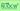# Perspectives in Mathematical Sciences IIILecturer Shigeru YAMAGAMI, Professor School of Science / Graduate School of Science, 2015 Spring 4th year students

### The Purpose of the Course

In his celebrated Erlangen Program in 1872, F. Klein opened a way to synthesize geometric objects based on group symmetry. Since then the notion of group has been playing significant roles in the study of various geometries. Among them, fundamental is the so-called projective geometry, which is intimately related to that of vector spaces. Interrelations of geometric positions of flat objects such as lines and planes in Euclidean spaces are described most aesthetically in the framework of projective geometry. The fundamental theorem of projective geometry then states that the three-point collinearity is enough to recover the linear group structure behind them. Its importance is not just restricted within purely mathematical subjects and we shall review here, in quantum theory and special relativity, two fundamentals in physics, how their symmetries can be realized as linear groups as applications of the fundamental theorem.

### The Method of Evaluation

Grading in this part is based on submitted reports on homeworks which will be assigned during the course. (No submission therefore means Nonattendance.)

Course notes will be provided at the first lecture time or you can directly refer to the source papers below.
 C.-A. Faure, An elementary proof of the fundamental theorem of projective geometry, Geom. Dedicata, 90(2002), 145-151.
 P.G. Vroegindewey, An algebraic generalization of a theorem of E.C. Zeeman, Indagationes Mathematica, 77(1974), 77-81.

### The Plan of the Course

Part 1 is scheduled to be 4/14, 4/21, 4/28, 5/12.

1. Review on affine spaces. 2. Touch of projective spaces. 3. The fundamental theorem of projective geometry. 4. Wigner's theorem on describing symmetry in quantum mechanics. 5. Alexandrov-Zeeman's theorem on describing symmetry in special relativity.

### Keywords

Projective geometry, affine geometry, symmetry in physics.

### Required Knowledge

Basic knowledge and skills in linear algebra and set theory.

### Attendance

This course is open for all students in Nagoya University as a part of open subject program. Certain amount of experience in the set-theoretic framework of mathematics is required, however, to get benefits from this part of the course.